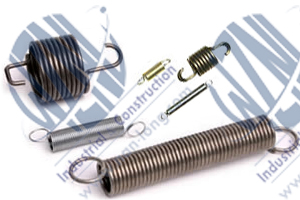# Extension Spring|ChinaSpring >>

# Extension SpringThere are two kinds of the hook of the extension spring. One is the english hook,and the othe is the german hook. The english hook is colsed one,and the german hook is not closed,and the german one is more endure than english one.According to customer's application requires,we could manufacture each kind.

# Extension spring (German Hook) parameters:Physical parameters :

• d (wire diameter): This parameter describes the diameter of wire used as material for spring.
• De (external diameter): External diameter of a spring concerns both hooks and coils.
• H (Hole): This is minimum diameter of hole in which spring can work.
• Ln (Maximum length): Maximum acceptable extension length for a spring. It is also the maximum extension for static applications.
• Fn (Maximal force): Maximal acceptable force which can operate on a spring. It is also the maximum force for static applications.
• L0 (free length): Free length is measured in spring's free state.
• Nr of coils: This is a total number of coils in a spring - in the picture above it is equal to six.
• R (Spring rate): This parameter determines spring's resistance, while it is working. It is measured in 1 DaN/mm = 10 N/mm.
• L1 & F1 (length under charge F): The charge F1 at length L1 can be calculated using the equation : F1 = Fn - R(Ln-L1). Equation derived from the previous for calculating L1 = Ln - (Fn-F1)/R.
• Hooks: Extension springs with german hooks are produced in two versions: with hook positions of 0 degrees and 90 degrees as shown in the picture above.
• Reference Number: All springs are identified by a unique reference: type . (De * 10) . (d * 100) . (L0 * 10) . material . [X if hooks are perpendicular to each other] ; for german extension springs, type is a letter "U", materials are identified by the following letters: "A" or "I". Example: U.063.090.0100.AX is an extension spring with an external diameter of 6.3 mm and a free length 10mm, produced from 0.9mm music wire with perpendicular hooks.

Extension spring (German Hook) parameters:Physical parameters :

• d (wire diameter): This parameter describes the diameter of wire used as material for spring.
• De (external diameter): External diameter of a spring concerns both hooks and coils.
• H (Hole): This is minimum diameter of hole in which spring can work.
• Ln (Maximum length): Maximum acceptable extension length for a spring. It is also the maximum extension for static applications.
• Fn (Maximal force): Maximal acceptable force which can operate on a spring. It is also the maximum force for static applications.
• L0 (free length): Free length is measured in spring's free state.
• Nr of coils: This is a total number of coils in a spring - in the picture above it is equal to six.
• R (Spring rate): This parameter determines spring's resistance, while it is working. It is measured in 1 DaN/mm = 10 N/mm.
• L1 & F1 (length under charge F): The charge F1 at length L1 can be calculated using the equation : F1 = Fn - R(Ln-L1). Equation derived from the previous for calculating L1 = Ln - (Fn-F1)/R.
• Hooks: Extension springs with English hooks have both hooks closed forming a ring. Both hooks lie in the same plane.
• Reference Number: All springs are identified by a unique reference: type . (De * 10) . (d * 100) . (L0 * 10) . material ; for english extension springs, type is a letter "T", materials are identified by the following letters: "A" or "I". Example: T.063.090.0100.A is the extension spring with the external diameter 6.3 mm and the free length 10mm, produced from 0.9 mm music wire .